# Kinematics of robot manipulators - forward kinematics 1

## (homogeneous transformation matrices)

Kinematics is the study of motion without reference to forces.  In engineering applications kinematics usually means finding the position (often called displacement), velocity and acceleration of a rigid body.  In these tutorials we are principally concerned with finding positions of the the robot links for a particular position of the tool tip in three dimensional space.  This position is often referred to as the tool tip pose.

In this series of tutorials we examine two approaches. The method of forward kinematics sets the position of each joint in turn and from this information computes the position of the tool tip. It is the subject of this tutorial and the next.

In later tutorials we examine inverse kinematics where we begin with the required position of the tool tip and then find the position of each link.  In practice inverse kinematics is more useful but we begin with forward kinematics as the analytic methods are more straightforward.

In this tutorial we show how robot links and co-ordinate systems are presented in a kinematic diagram and how angular movement and displacement of links are represented and calculated using a series of homogeneous transformation matrices.  I suggest reading the  first tutorial in the series If not familiar with basic terminology of robotics

A reasonable knowledge of linear algebra is necessary to follow some aspects of this tutorial.

#### Kinematic diagrams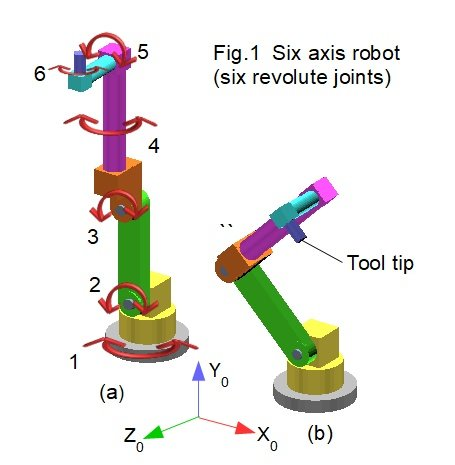In the  first tutorial we introduced the six axis robot shown in Fig.1 above, which is our model for kinematic analysis. Each link is shaded a different colour.  We use the physical construction of the robot to draw a kinematic diagram which defines co-ordinate systems (called frames) for each joint.

Firstly we define co-ordinates for the base or "real world" frame, shown on Fig.1*. This co-ordinate frame is indicated by subscript 0.  Its origin is at the centre of the top surface of the base plate (shaded grey). This is a "right hand" co-ordinate system (check images on-line if you are not familiar with the right-hand rule).   In the right hand rule counter-clockwise rotation about an axis is designated +ve.

* Co-ordinates are directly from the CAD model.  It is more conventional for Z to be the vertical co-ordinate in the base frame.

Each joint is assigned a co-ordinate frame located somewhere on its axis of rotation.  Co-ordinate frames form the basis of the kinematic diagram and the first task is to fix their position.

Figs.2 and 3 below show the construction of the robot model in a vertically extended pose with front view on the XY plane, and side view on the YZ plane.  Red dots show the chosen location of each frame for joints #1 to #6 and TT for the tool tip. The frame for joint 1 is coincident with the base frame.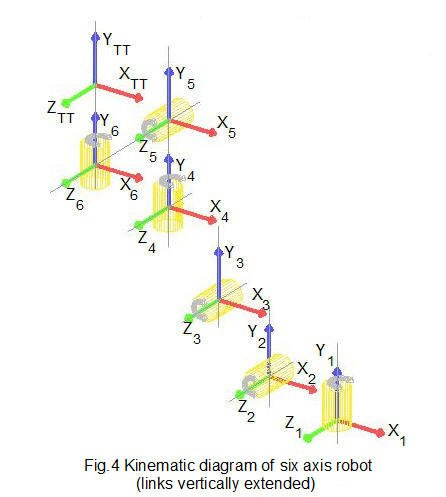Fig.4 shows co-ordinate frames in three dimensions for each joint in the vertically extended pose. Revolute joints are represented by cylinders . The diagram is not drawn to scale for clarity.  In this pose, orientations of X, Y and Z axes in each frame are chosen to be identical but this is not essential.  The origin of a frame can be at any convenient point on the axis of joint rotation and can even be outside the physical joint (as we shall see in the next tutorial).

We now add to the diagram the length and offset of each link.  The link length is the distance between successive frames on the longitudinal axis of the link*, not the external dimension of the link.  An offset occurs where the co-ordinate frames of two linked joints do not lie on the same axis.  In this pose the robot model has offsets on the Z axis only.

* Note the difference in determining link lengths associated with revolute joints type 1 or 2  as defined in the previous tutorial.

Fig.5 below shows link lengths and offsets on the kinematic diagram viewed on the YZ plane which provides a clearer view than the three dimensional diagram in Fig.4.  Again note that this diagram is not to scale.  Our convention is one subscript for link length, two for offset.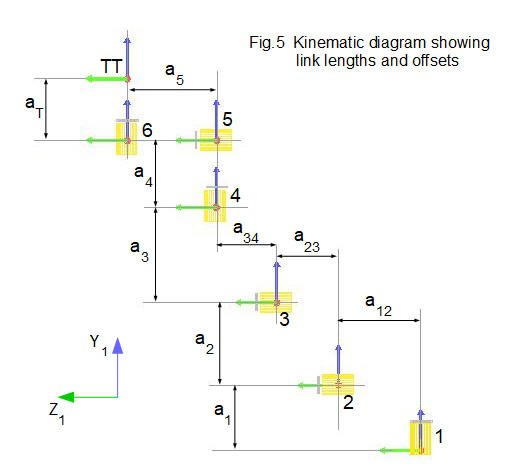The table below lists these dimensions in the robot model (unspecified length units).

 a1 a2 a3 a4 a5 aT a12 a23 a34 160 400 130 500 220 110 85 50 75

There is one more piece of information we require for forward kinematic analysis, namely the angle of rotation applied to each joint to achieve the tool tip pose shown in Fig.1(b).  In this example the joint rotations are:

• joint #2    rotate 45° counter-clockwise
• joint #3    rotate 90° clockwise
• joint #6    rotate 90° clockwise

The next section explains how we express movement of the robot mathematically using transformations of co-ordinate frames.

### Rotation and translation of co-ordinate frames

The objective of this kinematic analysis is to resolve the real world co-ordinates of the tool tip with reference to the base frame.  The robot model has six revolute joints in series. The co-ordinate frame for each joint can rotate about its joint axis and there are displacements between frames denoted by link lengths and offsets, where they exist.  Hence we need a method for resolving displacements in three dimensions that result from rotating the joints, starting at the base frame and finishing at the tool tip.

The most widely applied method which we outline below uses transformation matrices  to combine rotation and translation of co-ordinate frames (translation simply means linear displacement).  The following derivation of transformation matrices is presented in terms of vector transformations. The first part concerns rotation and the second part translation.

#### Rotation of co-ordinate frames

Fig.6 (a) and (b) show rotation of a right hand co-ordinate frame* about the Z axis.  We consider initially this rotation in two dimensions in the XY plane.

* It is conventional to use the notation X0 etc. for the base frame.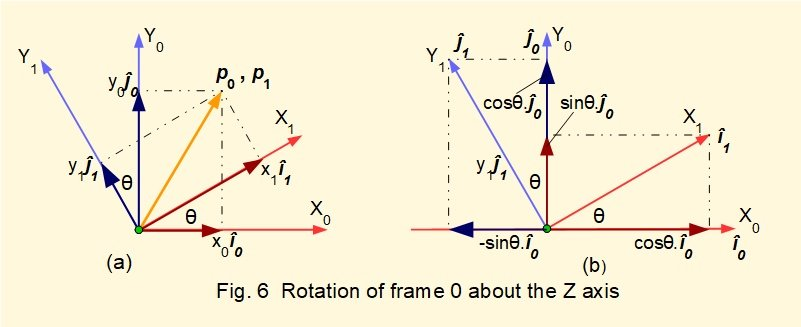Note: in the following text vectors are indicated in bold italic type.

Co-ordinate frame axes X0 and Y0 are rotated counter-clockwise by angle θ  to create a second frame 1 with axes X1 and Y1.  We envisage the axis of rotation coming out of the XY plane indicated by the green dot.

î0  ĵ0  î1 and ĵ1 are unit vectors on the X0, Y0, X1 and Y1 axes respectively and are represented in Fig.6b by the lengths of the axis arrows.

Fig 6a shows a position vector p0 for a point p in space with co-ordinates x0 and y0 in frame 0 .  The position vector for point p referred to rotated frame 1 is p1 with co-ordinates x1 and y1.  Note that p0p1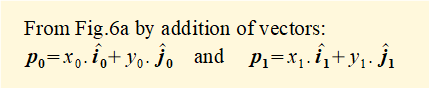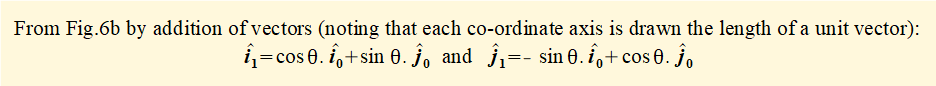Substituting the above expressions for î1 and ĵ1 into the above expression for p1 gives: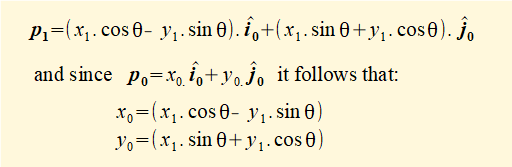We can state these equations in matrix form as: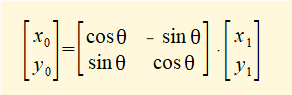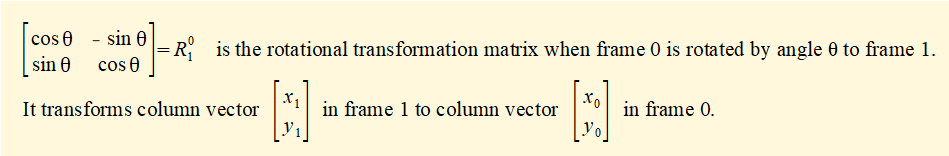Examining the columns of the matrix we see that columns 1 and 2 are respectively column vectors for: (i)  the projections of X1 on X0 and Y0 and (ii) the projections of Y1 on X0 and Y0, where X1 and Y1 are unit vectors.

We now expand the rotational matrix to three dimensions by introducing axes Z0 and Z1 and adding projections of X1 and Y1 on  Z0 and projections of Z1 on X0, Y0 and Z0.

In Fig.6 the Z axis is perpendicular out of the page hence projections of X1 and Y1 on Z0 = 0 and projections of Z1 on X0 and Y0 = 0.  The projection of Z1 on Z0 = 1 since the Z axis is unchanged by the rotation.  We can now expand the transformation matrix for rotation about the Z axis from two to three dimensions  as follows: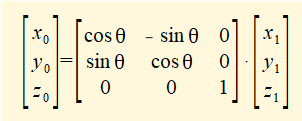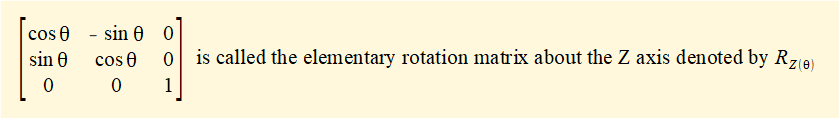The notation RZ(θ) indicates a rotation matrix for a rotation angle θ about the Z axis.

The corresponding elementary rotation matrices RX(θ) and RY(θ) for rotation about the X and Y axes respectively are: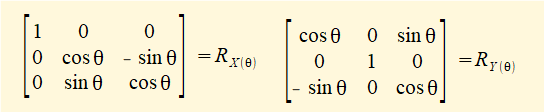Rotation matrices can be multiplied to obtain a transformation matrix for a series of frame rotations. Thus if the rotation of frame 0 to frame 1 about axis Z0 is angle θ and the rotation of frame 1 to frame 2 about axis Z1 is angle φ then: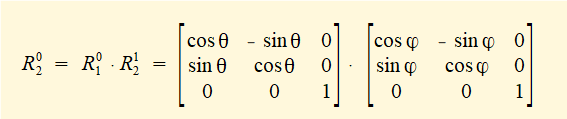The reverse rotational transformation matrix from frame 1 to frame 0 is the transpose of R01, i.e.R10 = (R01)T

#### Translation of co-ordinate frames

By translation we mean moving the origin of a co-ordinate frame to a new point in space   In the context of forward kinematics the new point is the origin of the co-ordinate frame for the next joint.

Clearly it is useful to combine translation and rotation in one transformation matrix.  We now show how this is done, initially in two dimensions and then expanded to three dimensions.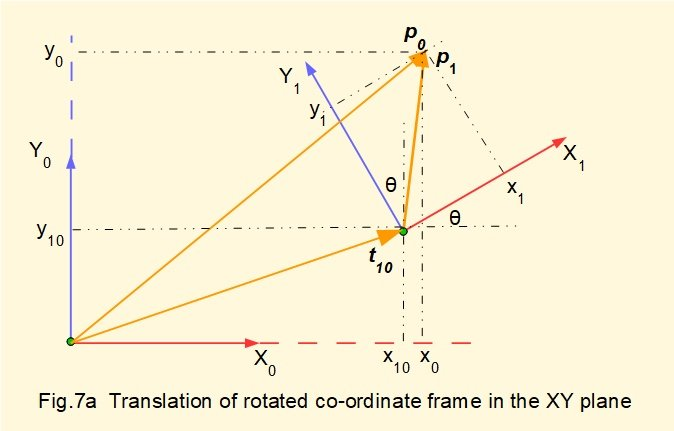Figs.7a and 7b show rotated co-ordinate frame 1 translated in the XY plane from its original position coincident with the origin of frame 0.

In Fig 7a consider point p in space represented by position vector p1 in frame 1 with co-ordinates x1 and y1.  Point p is also represented by position vector p0 in frame 0. However, vectors p1 and p0 cannot be added legitimately because co-ordinate frames 0 and 1 are not parallel. Vector t10 is the position vector of the origin of frame 1 in frame 0.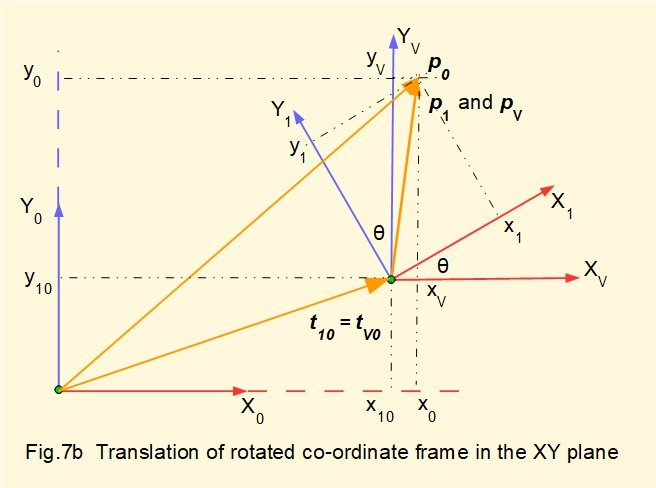In Fig 7b introduce a co-ordinate frame V which is parallel to frame 0 and shares its origin with frame 1.  Point p is now represented by position vector pV in frame V.  Note that pVp1. The origin of frame V with respect to frame 0 is represented by vector tV0 which is equal to vector t10

Because frame V is parallel to frame 0 we can add vectors in frames 0 and V such that    p0 = tV0 + pV   ................ (i)

Now we replicate frame 1 by rotating frame V by angle θ, using rotational transformation matrix RV1

which  gives :   pV = RV1 . p1  ................... (ii)

substituting for pV in (i) gives:     p0 = tV0 + RV1 . p1

now RV1 = R01 (equal rotations of angle θ) and tV0 = t10

giving      p0 = t10 + R01 . p1  ..................... (iii)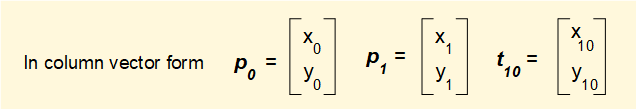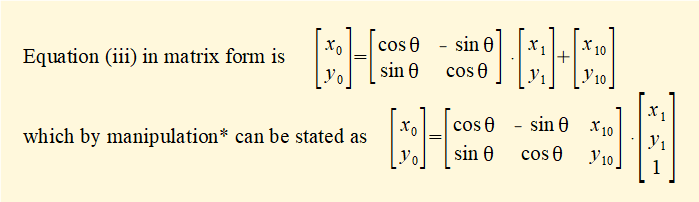* this step is not obvious but is easily checked by expanding dot products in both equations.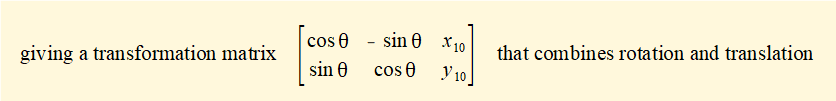*  Homogeneous co-ordinates add one dimension to Cartesian co-ordinates and are widely used for linear transformations in computing.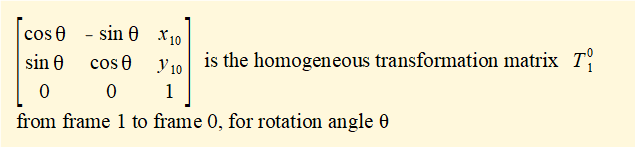Note that the homogeneous transformation matrix takes the form: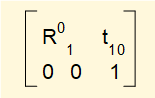where R01 is the rotational transformation matrix and t10 is the column vector representing translation of the origin of frame 1 to the origin of frame 0.

The reverse homogeneous transformation matrix for a transformation from frame 0 to frame 1 is the inverse of T01, i.e.T10 = (T01)-1 (not the transpose as for R01 reversed)

Homogeneous transformation matrices can be multiplied to obtain a transformation matrix for a series of frame rotations and translations such that T02 = T01 • T12

We now extend the homogeneous transformation matrix for rotation and translation of co-ordinate frames to three dimensions illustrated in Fig.8.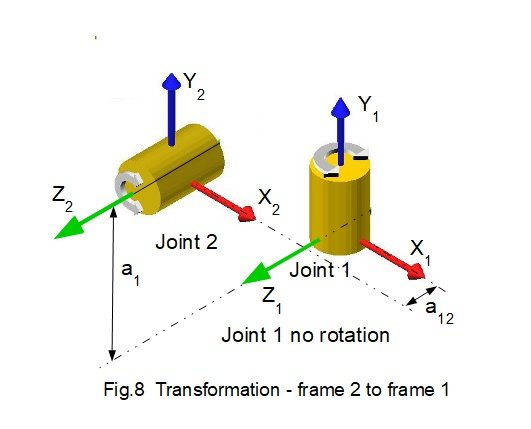Derivation of the homogeneous transformation matrix for three dimensions is broadly identical to the two dimensional case using homogeneous vectors with X, Y and Z components.  The result is a [4 x 4] homogeneous transformation for rotation about the Z axis as follows.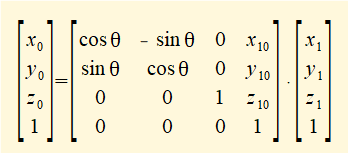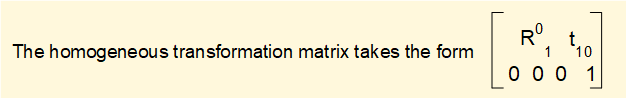Where R01 is the elementary rotation matrix and t10 is the column vector representing translation of the origin of the co-ordinate frame, shown in Fig.8.  We call the vector t10 the translation (or displacement) vector.

The homogeneous transformation matrices for rotation about the X, Y and Z axes are thus: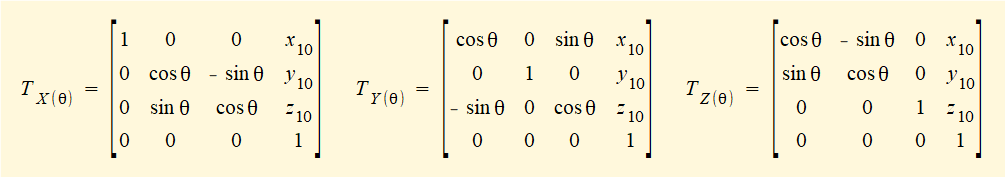As with the two dimensional case we can combine homogeneous transformations to obtain a transformation matrix for a series of frame rotations and translations such that T0n = T01 • T12  • ----- •T(n-1) n.   Examination of the dot product shows that the translation vector part  tn0 of matrix T0n expresses the co-ordinates of the origin of frame n  with respect to frame 0.

We now have a mathematical tool for forward kinematic analysis of the robot model.  The next section of the tutorial examines each joint of the robot in sequence, applying the homogeneous transformation matrix at each stage.

### Frame by frame analysis

Commercial software (for example  MATLAB) computes multiple transformations using joint angles, link lengths and offsets as input variables. For our purpose, however, it is instructive to compute each transformation using a matrix calculator, find the real world co-ordinates of each joint and then validate the output directly from the CAD robot model.

#### Transformation - frame 1 and frame 2

Fig.9 is an enlarged section of the kinematic diagram in Fig.3  showing joints 1 and 2  connected by link 1.  Joint 1 is a revolute joint with rotation about the Y axis.  The initial state of frame 1 has co-ordinates  x1 = 0,  y1 = 0,  z1 = 0 (i.e. identical to the co-ordinates of the base frame).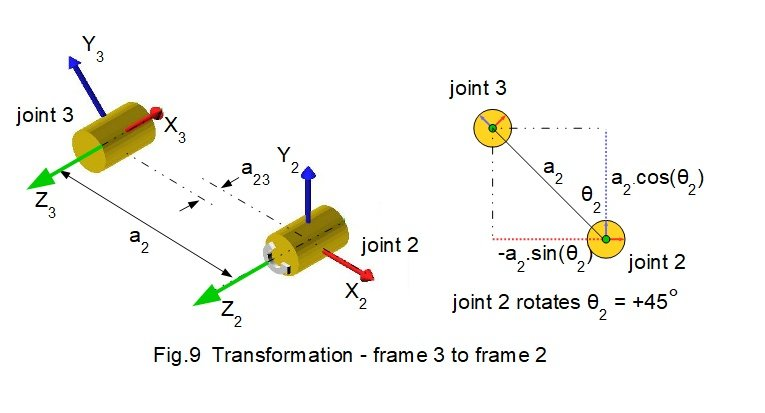Parameters for transformation T12 are:

• there is no rotation of frame 1.  Thus θ1 = 0°
• frame 1 translates to frame 2 along link length a1 on the Y axis and link offset a12 on the Z axis.
• link length a1 = 160
• link offset a12  = 85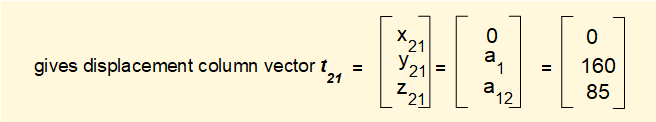Inserting these values into the homogeneous* transformation matrix for rotation about the Y axis gives:

* from this point transformation matrix refers to the homogeneous version.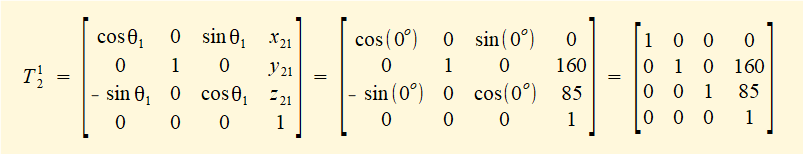Note that the rotational part of the transformation matrix in the upper left hand section is the identity matrix.  This is consistent with no rotation.

We use the transformation matrix T12 to state co-ordinates x2, y2, z2 within frame 2 in terms of co-ordinates x1, y1, z1 within frame1 from the equation: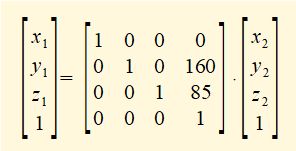We want to express co-ordinates of the origin of frame 2 in terms of frame 1. Thus:  x2 = 0, y2 = 0, z2 = 0 giving: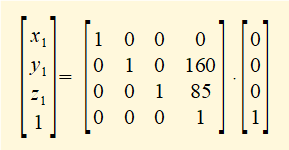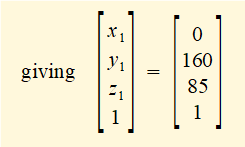Note that the translation vector t21 in the transformation matrix yields the required real world co-ordinates.  This result was predictable since frame 2 does not rotate relative to the base.  Examining the above dot product in fact reveals that the translation vector tn1 yields the required result for transformations to frame 1 from the origin of each frame n.

.

#### Transformation - frame 2 and frame 3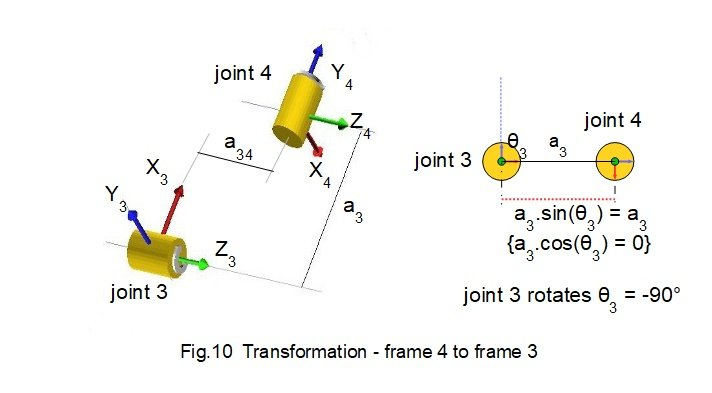For joint rotation θ2 the projections of a2 are a2.cosθ2 on the Y axis and - a2.sinθ2 on the X axis, where a2 is the link length. This is determined by the datum position for rotations in the XY plane being aligned with the Y axis*.

* It is more conventional to set the datum for kinematic analysis aligned with the X axis.  For this example a vertical datum provides clearer diagrams.

Parameters for transformation T23 are:

• frame 2 rotates  45° in a positive (counter-clockwise) direction.  Thus  θ2  = 45°
• frame 2 translates to frame 3 along link length a2 and link offset a23 on the Z axis.
• link length a2 = 400
• link offset a23 = 50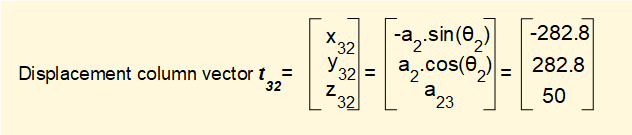Inserting these values into the transformation matrix for rotation about the Z axis gives: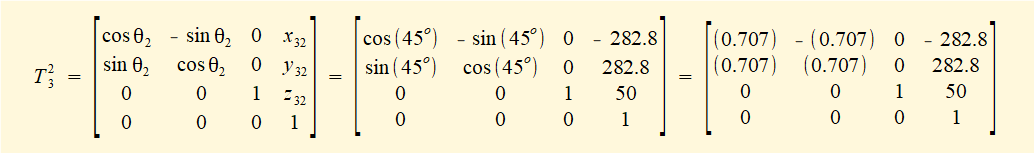We now perform the matrix multiplication T13 =  T12 • T23 giving: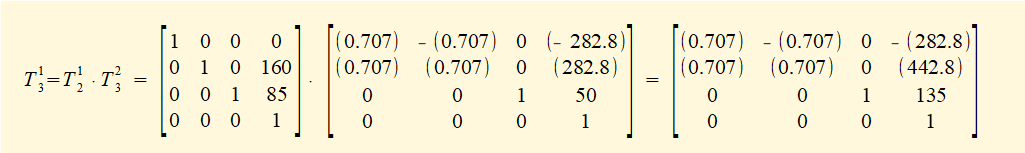Real world co-ordinates for the origin of frame 3 after the transformation read from the t31 section of matrix T13 are as follows: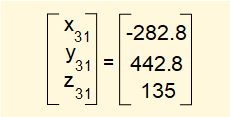#### Transformation - frame 3 and frame 4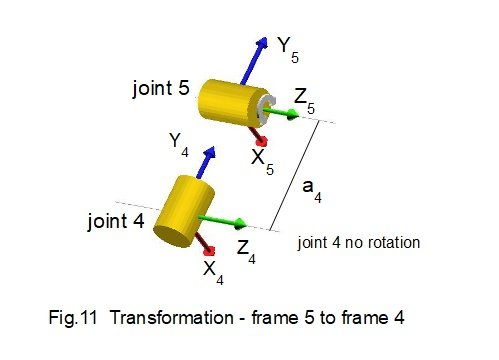To give a better perspective the viewpoint of Fig.11 has changed from previous diagrams.

Parameters for transformation T34 are:

• frame 3 rotates 90° in a negative (clockwise) direction.  Thus θ3  =  -90°
• frame 3 translates to frame 4 along link length a3 and link offset a34 on the Z axis.
• link length a3 = 130
• link offset a34 = 75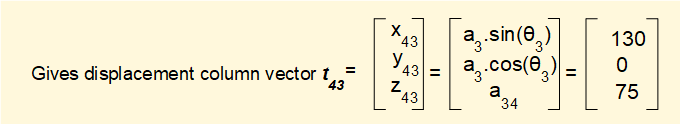Inserting these values into the transformation matrix for rotation about the Z axis gives: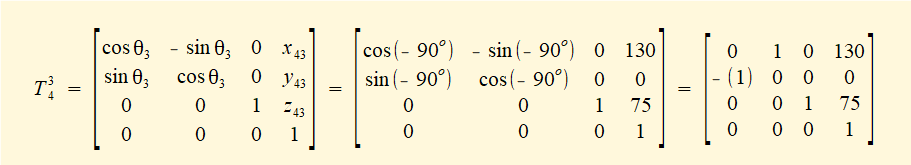We now perform the matrix multiplication T14 =  T12 • T23 • T34  giving: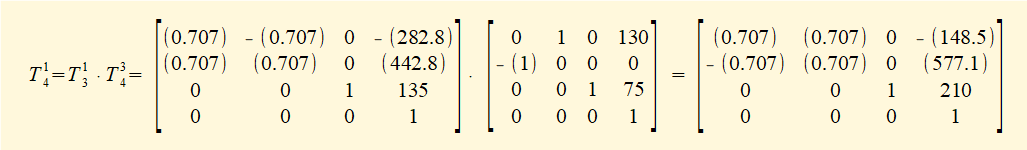Real world co-ordinates for the origin of frame 4 after the transformation read from the t41 section of matrix T14 are as follows: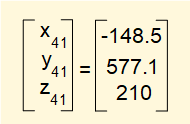#### Transformation - frame 4 and frame 5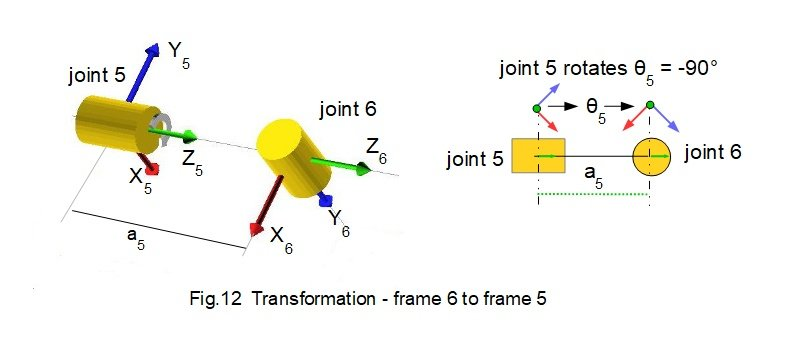Parameters for this transformation T45 are:

•  there is no rotation.  Thus θ4  = 0°
• frame 4 translates to frame 5 along link length a4. There is no link offset on the Z axis.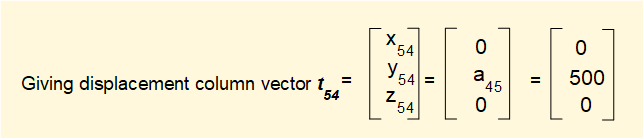Inserting these values into the transformation matrix for rotation about the Y axis gives: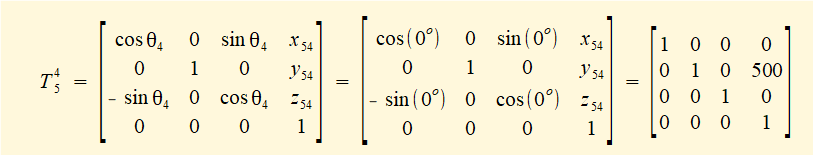We now perform the matrix multiplication T15 =  T12 • T23 • T34 • T45   giving: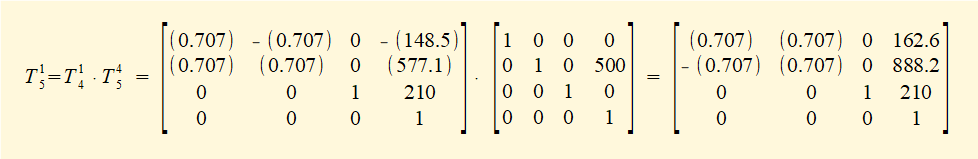Real world co-ordinates for the origin of frame 5 after the transformation read from the t41 section of matrix T14 are as follows: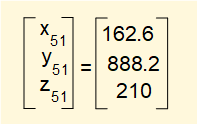#### Transformation - frame 5 and frame 6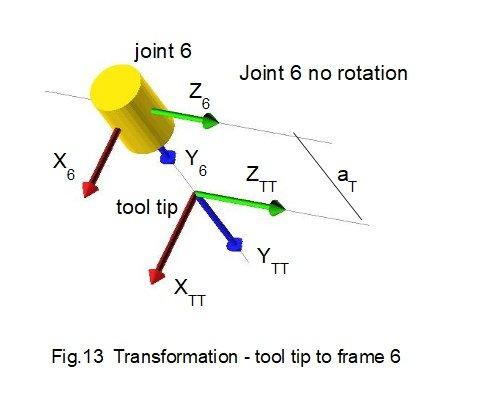Parameters for this transformation T56 are:

• frame 5 rotates 90° in a negative (clockwise) direction about the Z axis.  Thus θ5  =  -90°
• frame 5 translates to frame 6 along link length a5 on the Z axis.
• link length a5 = 220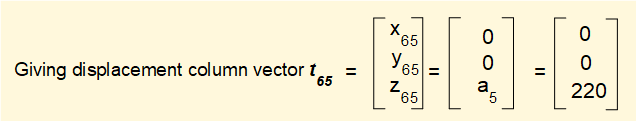If you are unsure why components  x65 = 0 and y65 = 0 consider that the projection of the origin of rotated frame 6 on frame 5 passes through the origin of frame 5 (for further illustration, in Fig.7 consider the projection of the origin of frame 1 on the Y0 axis  if translation of frame 1 were along the X0 axis only).

Inserting these values into the transformation matrix for rotation about the Z axis gives: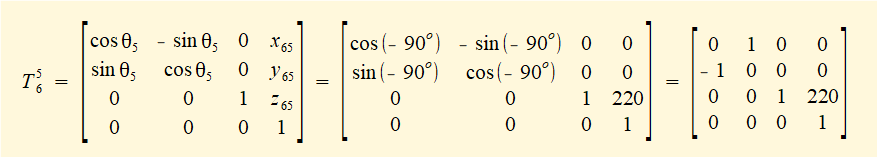We now perform the matrix multiplication T16 =  T12 • T23 • T34 • T4• T56  giving:Real world co-ordinates for the origin of frame 6 after the transformation read from the t61 section of matrix T16 are as follows: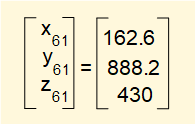#### Transformation - frame 6 and frame TT (tool tip)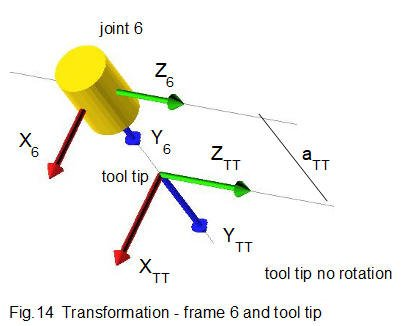Parameters for transformation T6TT are:

• there is no rotation.  Thus θ6  = 0°
• frame 6 translates to frame TT along link length aTT  on the Y axis.
• link length aTT = 110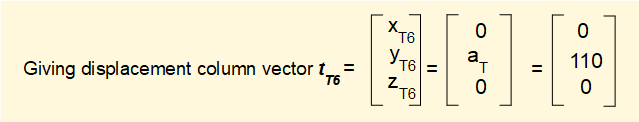No rotation is equivalent to placing  the identity matrix in the rotation section of the transformation matrix  Thus T6TT  can be stated as: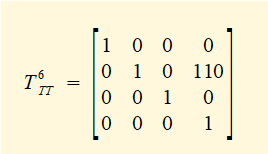We now perform the (final!) matrix multiplication T1TT =  T12 • T23 • T34 • T4• T56 • T6TT   giving: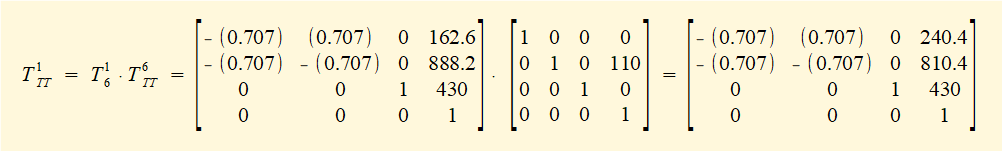Real world co-ordinates for the tool tip after the transformation read from the tT1 section of matrix T1TT are as follows: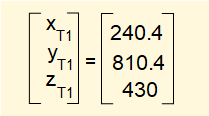We have completed the forward kinematic analysis using homogeneous transformations.  In the next tutorial we explain another method using transformation matrices known as the Denavit Hartenberg method.

I welcome feedback at:

### Tutorials - mechanical vibrations

###### Forced vibrations with damping

Alistair's tutorials 2021